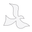If you want to find out your size at home, just have a flexible measuring tape. This is placed around the head above the ears, at the height of the middle of the forehead and the most protruding in the back. This would be the level where the hat normally rests. The tape measure should be taught, but not tight. Make a note of the centimeter length. In case you don't have a flexible measuring tape, we  have a trick: you can use a thread and then measure it with a ruler.

Equivalence

7        (Size S)    = 56 cm =  22 inches   US

7 1/8 (Size M)  = 57 cm =  22.4 inches US

7 1/4 (Size L)   = 58 cm =  22.8 inches US

7 3/8 (Size XL)  = 59 cm =  23.2 inches US

7 1/2 (Size XXL) = 60 cm =  23.6 inches US